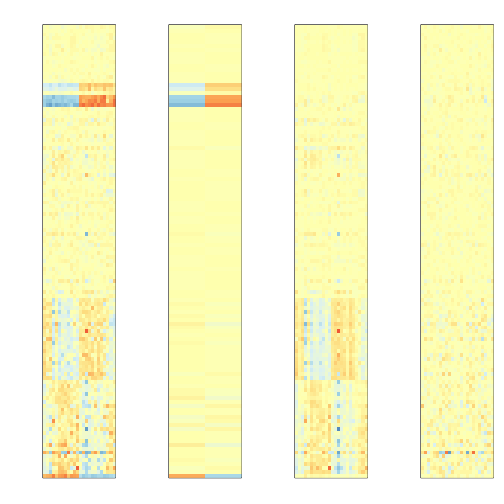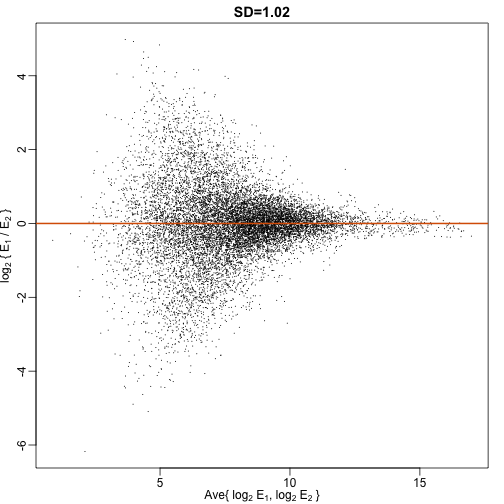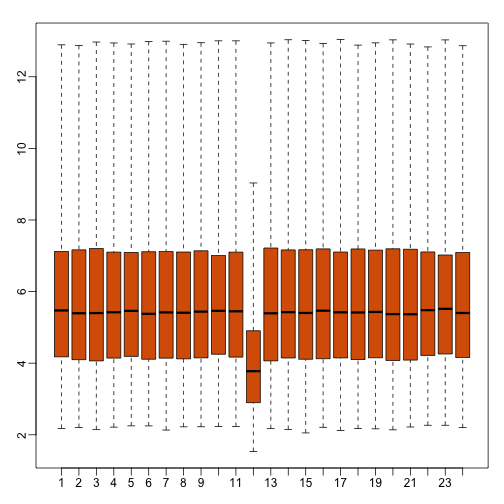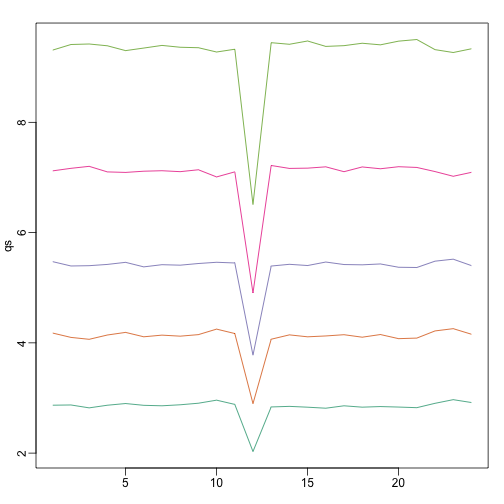# 理解基因组数据分析之建模与可视化篇

$$Y = \alpha * X + \beta$$

Y是响应，假设有两组，我们给组A赋值1，组B赋值0，那么系数$\beta$就是组B的均值而系数$\alpha$则是组A与组B的差值。这个差值实际上就是t检验里的差异，对这个系数的估计出的t值就是t检验的t值。

# 模拟生成两组数据

set.seed(42)

group1 <- rnorm(100,100,10)

group2 <- rnorm(100,130,13)

# 进行t检验

t.test(group1,group2)

##

## Welch Two Sample t-test

##

## data:  group1 and group2

## t = -18.173, df = 195.17, p-value < 2.2e-16

## alternative hypothesis: true difference in means is not equal to 0

## 95 percent confidence interval:

##  -31.63463 -25.44050

## sample estimates:

## mean of x mean of y

##  100.3251  128.8627

# 构建分组变量

fac <- c(rep(0,100),rep(1,100))

# 构建数据变量

dat <- c(group1,group2)

# 回归分析

fit <- lm(dat~fac)

summary(fit)

##

## Call:

## lm(formula = dat ~ fac)

##

## Residuals:

##     Min      1Q  Median      3Q     Max

## -30.256  -6.523   0.566   6.532  36.262

##

## Coefficients:

##             Estimate Std. Error t value Pr(>|t|)

## (Intercept)   100.33       1.11   90.35   <2e-16 ***

## fac            28.54       1.57   18.17   <2e-16 ***

## ---

## Signif. codes:  0 '***' 0.001 '**' 0.01 '*' 0.05 '.' 0.1 ' ' 1

##

## Residual standard error: 11.1 on 198 degrees of freedom

## Multiple R-squared:  0.6252,Adjusted R-squared:  0.6233

## F-statistic: 330.2 on 1 and 198 DF,  p-value: < 2.2e-16

$$Y = \alpha * X + \gamma * Year + \beta$$

# 安装bioconductor

source("http://bioconductor.org/biocLite.R")

biocLite()

# 安装绘图用的rafalib包跟RcolorBrewer包还有示例的GSE5859Subset数据集

# install.packages('rafalib')

# install.packages('RColorBrewer')

# devtools::install_github("genomicsclass/GSE5859Subset")

# genefilter包用来同时进行高维数据操作

# biocLite('genefilter')

# 加载软件包与数据集

library(GSE5859Subset)

library(rafalib)

library(genefilter)

library(RColorBrewer)

# GSE5859Subset数据集收集了不同人种的基因表达数据的一部分

data(GSE5859Subset)

# 按照性别分组

sex <- sampleInfo$group # 提取混杂变量时间 batch <- factor(format(sampleInfo$date,"%m"))

# 提取染色体编号

chr <- geneAnnotation$CHR # 对不同时间进行t检验观察混杂是否存在 tt<-rowttests(geneExpression,batch) # 提取Y染色体 ind1 <- which(chr=="chrY") ##real differences # 提取时间尺度上不在Y染色体上的变化最大的50个基因中 ind2 <- setdiff(c(order(tt$dm)[1:25],order(-tt$dm)[1:25]),ind1) # 从上面两组基因之外再随机选50个基因 set.seed(1) ind0 <- setdiff(sample(seq(along=tt$dm),50),c(ind2,ind1))

# 这三组基因提取的各代表一定有变化的（Y染色体女性没有），采样时间导致的变化以及其他随机找的50个基因

geneindex<-c(ind2,ind0,ind1)

# 截取相应数据

mat<-geneExpression[geneindex,]

# 归一化

mat <- mat -rowMeans(mat)

# 绘图

mypar(1,2)

# 所有截取的数据

icolors <- colorRampPalette(rev(brewer.pal(11,"RdYlBu")))(100)

image(t(mat),xaxt="n",yaxt="n",col=icolors)

# 所有数据归一化

y <- geneExpression - rowMeans(geneExpression)

# 绘制样本间分性别相关趋势图

image(1:ncol(y),1:ncol(y),cor(y),col=icolors,zlim=c(-1,1),

xaxt="n",xlab="",yaxt="n",ylab="")

axis(2,1:ncol(y),sex,las=2)

axis(1,1:ncol(y),sex,las=2)# 安装相关软件包sva与limma

# biocLite(c('sva','limma'))

# 读取进行替代变量分析与回归的sva跟limma包

library(limma)

library(sva)

# 构建基础模型

mod <- model.matrix(~sex)

# 寻找替代变量

svafit <- sva(geneExpression,mod)

## Number of significant surrogate variables is:  5

## Iteration (out of 5 ):1  2  3  4  5

# 构建包含替代变量的模型

svaX<-model.matrix(~sex+svafit$sv) # 拟合模型 lmfit <- lmFit(geneExpression,svaX) # 提取替代变量矩阵 Batch<- lmfit$coef[geneindex,3:7]%*%t(svaX[,3:7])

# 提取效应矩阵

Signal<-lmfit$coef[geneindex,1:2]%*%t(svaX[,1:2]) # 提取误差矩阵 error <- geneExpression[geneindex,]-Signal-Batch # 归一化作图 Signal <-Signal-rowMeans(Signal) mat <- geneExpression[geneindex,]-rowMeans(geneExpression[geneindex,]) mypar(1,4,mar = c(2.75, 4.5, 2.6, 1.1)) image(t(mat),col=icolors,zlim=c(-5,5),xaxt="n",yaxt="n") image(t(Signal),col=icolors,zlim=c(-5,5),xaxt="n",yaxt="n") image(t(Batch),col=icolors,zlim=c(-5,5),xaxt="n",yaxt="n") image(t(error),col=icolors,zlim=c(-5,5),xaxt="n",yaxt="n")可以看出，通过替代变量分析我们最大程度上保留了原有效应并剔除了替代变量。在这个基础上配合前文提到的经验贝叶斯方法进行基因筛选，得到的基因为我们比较感兴趣的部分了，下一篇将在此基础上讨论如何对结果进行注释或者基因组序列比对。 在进行下一部分之前，我们有必要讨论下一些基因组数据分析的可视化方法，这些方法不见得新，但有利于我们发现数据本身的问题而不是蒙着头只凭过往经验做分析。 可视化 基因组数据的结构决定其可视化必然要适应高维属性并面向实际问题。下面分问题阐述且前面提到的火山图与热图就不单独介绍了： 重复性问题 你进行了一组技术重复，然后发现两组数据相关性不错，那么是不是数据就可信了？不一定，第一次你测到是1，3，5，7但第二次测到的是10，30，50，70。这种情况相关性非常好，但都差了一个数量级。我们用数据做个演示。 # biocLite("SpikeInSubset") library(SpikeInSubset) # 载入原始数据 data(mas95) # 提取表达谱信息并用散点图绘制 mypar(1,2) r <- exprs(mas95)[,1] ##original measures were not logged g <- exprs(mas95)[,2] plot(r,g,lwd=2,cex=0.2,pch=16, xlab=expression(paste(E)), ylab=expression(paste(E)), main=paste0("corr=",signif(cor(r,g),3))) abline(0,1,col=2,lwd=2) # 寻找95%的数据集中的部分 f <- function(a,x,y,p=0.95) mean(x<=a & y<=a)-p a95 <- uniroot(f,lower=2000,upper=20000,x=r,y=g)$root

abline(a95,-1,lwd=2,col=1)

text(8500,0,"95% of data below this line",col=1,cex=1.2,adj=c(0,0))

# 在对数范围上重新绘图

r <- log2(r)

g <- log2(g)

plot(r,g,lwd=2,cex=0.2,pch=16,

xlab=expression(paste(log, " ", E)),

ylab=expression(paste(log, " ", E)),

main=paste0("corr=",signif(cor(r,g),3)))

abline(0,1,col=2,lwd=2)mypar(1,1)

plot((r+g)/2,(r-g),lwd=2,cex=0.2,pch=16,

xlab=expression(paste("Ave{ ",log, " ", E,", ",log, " ", E," }")),

ylab=expression(paste(log," { ",E," / ",E," }")),

main=paste0("SD=",signif(sqrt(mean((r-g)^2)),3)))

abline(h=0,col=2,lwd=2)ge <- geneExpression

# 伪造一组有问题的数据

ge[,12] <- ge[,12]/log2(exp(1))

mypar(1,1)

# 用boxplot检查

boxplot(ge,range=0,names=1:ncol(ge),col=ifelse(1:ncol(ge)==42,1,2))# 用matplot绘制分位数曲线

qs <- t(apply(ge,2,quantile,prob=c(0.05,0.25,0.5,0.75,0.95)))

matplot(qs,type="l",lty=1)# 绘制平滑直方图

shist(ge,unit=0.5)http://wap.sciencenet.cn/blog-430956-917067.html

## 相关博文

GMT+8, 2021-7-30 01:29